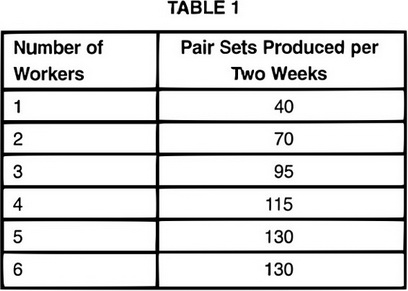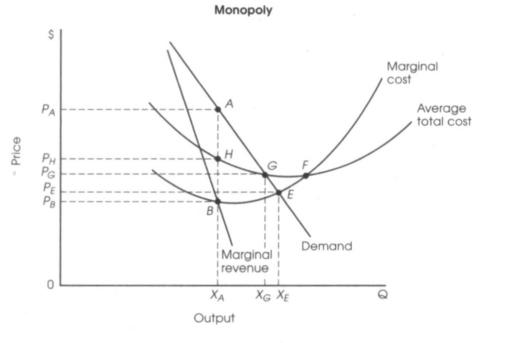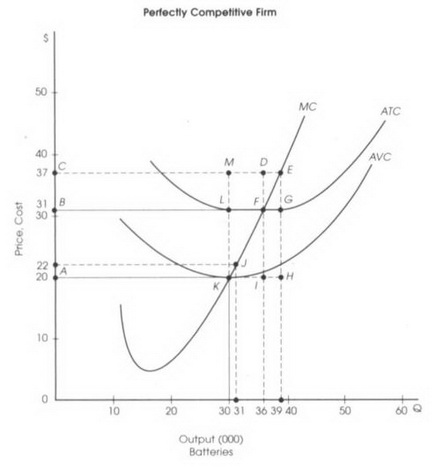# AP Microeconomics Practice Test 7

### Test Information12 questions14 minutes

1. Which of the following is true about a price ceiling?

2. Which of the following situations best exemplifies the concept of consumer surplus?

3.Given the data in the table above, and knowing that workers are paid \$1,250 every two weeks and that the pair sets are sold to retailers at \$50, how many workers would be hired?

4.An unregulated monopoly would

5.A regulated monopoly could occur at

6.If regulation of a natural monopoly occurs where marginal cost intersects the demand curve, which of the following is correct?

7.Which of the following is correct about the profits of an unregulated monopolist?

8.At a market price of \$37, maximum profits are

9.At a market price of \$19, how many batteries will the firm produce?

10.At a market price of \$22, how many batteries will the firm produce?

11.At the market price of \$22, the output will result in

12. If the government announces that the drinking of red grape juice reduces the risk of heart attacks, which of the following will be correct in terms of changes in quantity demanded, demand, and the price of red grape juice?

Quantity demanded      Demand      Price of grape juice